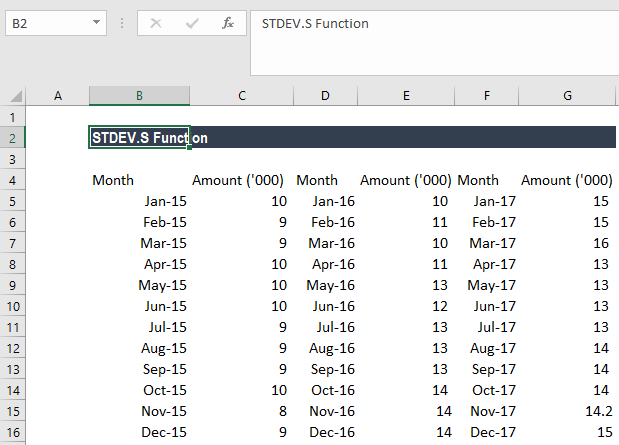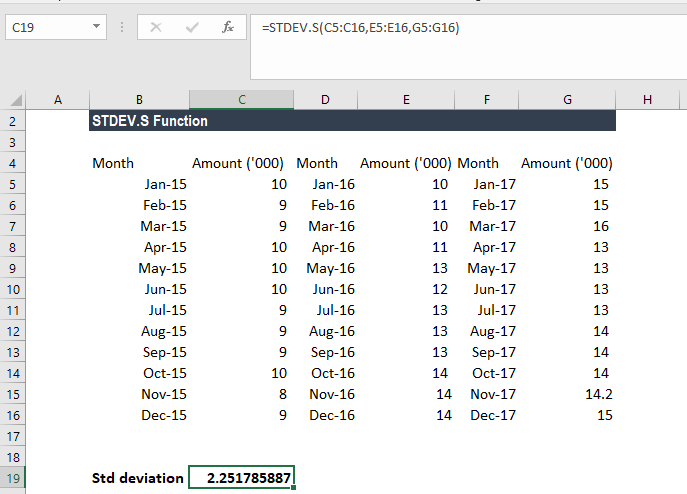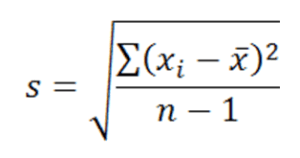# STDEV.S Function

Standard deviation for a sample of the population

## What is the STDEV.S Function?

The STDEV.S Function is an Excel Statistical function that will calculate the standard deviation that is based on a sample of the population. It will ignore logical values and text.

While doing financial analysis, the STDEV.S function can be useful in, for example, measuring the income standard deviation representative of an entire population.

### Formula

=STDEV.S(number1,[number2],…)

The STDEV.S function uses the following arguments:

1. Number1 (required argument) – This is the first number argument that corresponds to a sample of the population.
2. Number2 (optional argument) – This is a number argument that corresponds to a second sample of the population.

#### Notes

1. The given arguments must provide at least two numeric values to the function.
2. Arguments can either be numbers or names, arrays, or references that contain numbers.
3. The STDEV.S function is used to calculate the SD of a sample and not of a whole population. To calculate the standard deviation for a population, use either the STDEVP function or the STDEV.P function.

### How to use the STDEV.S Function in Excel?

As a worksheet function, STDEV.S can be entered as part of a formula in a cell of a worksheet. To understand the uses of the function, let us consider an example:

#### Step by Step Example of STDEV.S Function

Suppose we are given a record of a business’ monthly expense figures over the last three years, as shown below:For the standard deviation, the formula used is:We get the results below:The equation used for this is:Where:

• takes on each value in the set
• x is the average (statistical mean) of the set of values
• is the number of values

### Things to remember about the STDEV.S Function

1. #DIV/0! error – Occurs if none of the values that are supplied to the function is numeric.  Sometimes the number may not be selected as a number.
2. #VALUE! error – Occurs if any input values in the STDEV function are values that aren’t numeric values.
3. The STDEV.S function is used when calculating the standard deviation for an entire population. If we are calculating the standard deviation of an entire population, we need to use the STDEV.P function.

Thank you for reading this CFI guide to important Excel functions! By taking the time to learn and master these formulas, you’ll significantly speed up your financial modeling and analysis. To learn more, check out these additional CFI resources:

• Excel for Finance
• INDEX MATCH
• OFFSET
• Shortcuts

### Free Excel Tutorial

To master the art of Excel, check out CFI’s FREE Excel Crash Course, which teaches you how to become an Excel power user.  Learn the most important formulas, functions, and shortcuts to become confident in your financial analysis.

Launch CFI’s Free Excel Course now to take your career to the next level and move up the ladder!